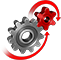# WolframSystemModeler

## A Pendulum Dangling from a Slider-Crank

How can you use Wolfram System Modeler to model a pendulum dangling from a slider-crank?

To run this example, you'll need

### The Dangling Pendulum Model

The crank wheel has a fixed translational position and a set rotational speed that drives the rest of the system. The pendulum joint has to be modeled in a certain way in order for its y position to be locked while simultaneously allowing both rotational movement and translational movement in the x direction.
The model as seen in the diagram view in Wolfram System Modeler. The pendulum joint is modeled with a prismatic joint, for the ability to lock its y position and allow movement along the x axis, and two revolute joints. Two revolute joints are needed to get the correct degrees of freedom.

### Simulation and Animation

By extracting the variables describing the movement of the different mechanical parts, Wolfram System Modeler and the Wolfram Language can be combined to create an interactive visualization of the dangling pendulum.Change the settings of the dangling pendulum and see how it behaves.

Create model‐based visualizations

The dangling pendulum is well suited for animation in Mathematica, where the movement of different mechanical parts can be obtained directly from System Modeler simulation results.

## Wolfram System Modeler

Questions? Comments? Contact a Wolfram expert »